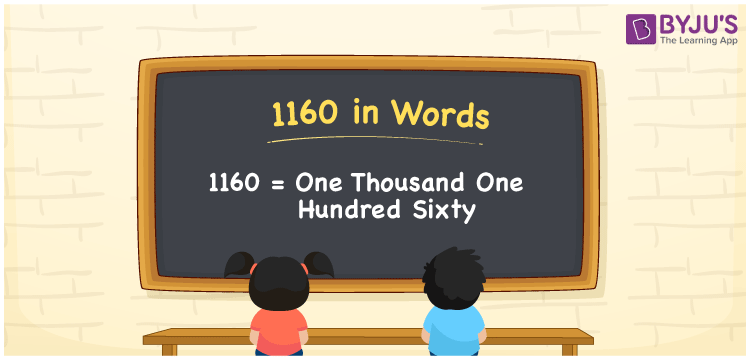# 1160 in Words

In English words, the number 1160 represents one thousand one hundred sixty. 1160 is a cardinal number as it is used to count the object which is equivalent to one thousand one hundred sixty. For example, the cost of 10 pens is Rs. 1160. The place value system plays a major role in converting the number 1160 into words.

 1160 in Words: One Thousand One Hundred Sixty. One Thousand One Hundred Sixty in Numerical Form: 1160.

## 1160 in English Words## How to Write 1160 in Words?

The number 1160 is a four-digit number, and its place value chart is represented below:

 Thousands Hundreds Tens Ones 1 1 6 0

The expanded form of 1160 is as follows:

= 1 × Thousand + 1 × Hundred + 6 × Ten + 0 × One

= 1 × 1000 + 1 × 100 + 6 × 10

= 1000 + 100 + 60

= 1160

= one thousand one hundred sixty

Hence, 1160 in words is one thousand one hundred sixty.

1160 in words – One thousand one hundred sixty

Is 1160 an odd number? – No

Is 1160 an even number? – Yes

Is 1160 a perfect square number? – No

Is 1160 a perfect cube number? – No

Is 1160 a prime number? – No

Is 1160 a composite number? – Yes

## Frequently Asked Questions on 1160 in Words

Q1

### How to spell 1160?

1160 in words is one thousand one hundred sixty.

Q2

### Simplify 1000 + 160, and express it in words.

Simplifying 1000 + 160, we get 1160. Hence, 1160 in words is one thousand one hundred sixty.

Q3

### Is 1160 a prime number?

No, 1160 is not a prime number.

Test your Knowledge on 1160 in Words